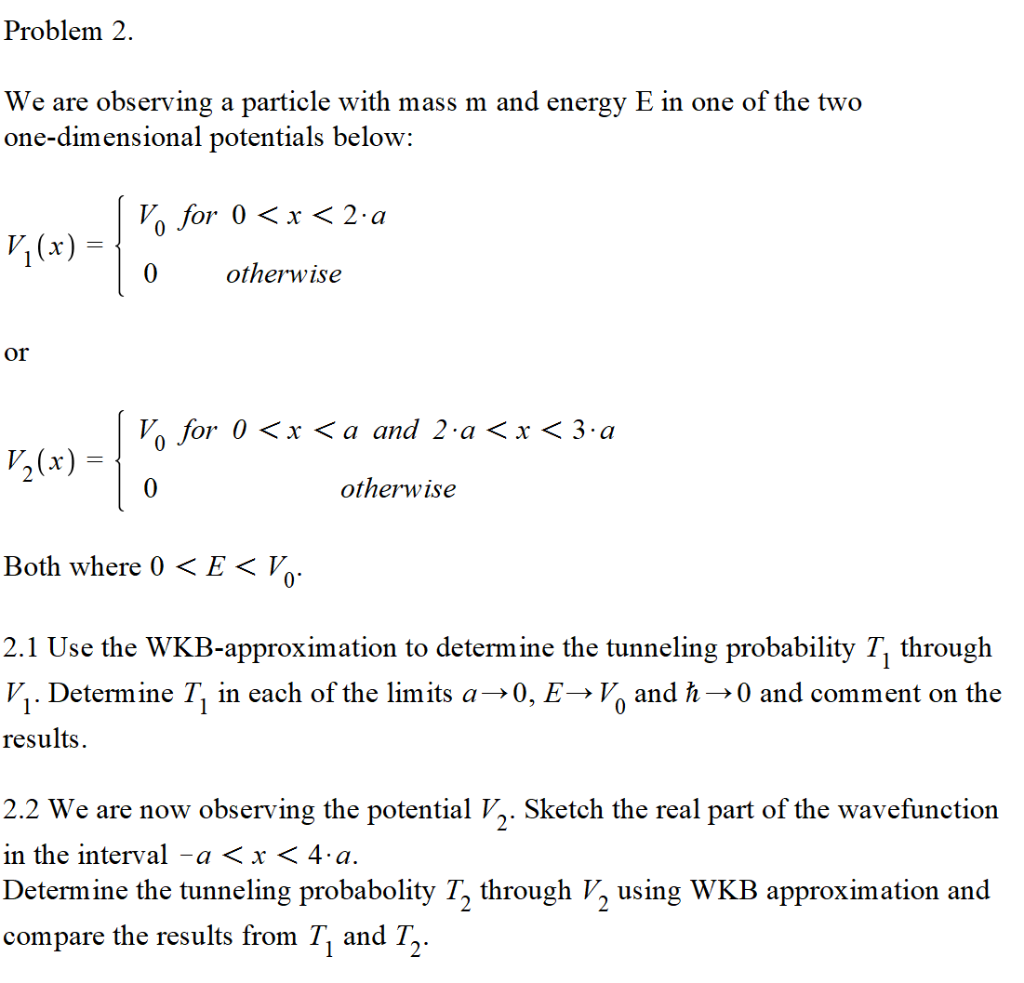Home / Answered Questions / Other / problem-2-we-are-observing-a-particle-with-mass-m-and-energy-e-in-one-of-the-two-one-dimensional-pot-aw424

# (Solved): Problem 2. We Are Observing A Particle With Mass M And Energy E In One Of The Two One-dimensional Po...Problem 2. We are observing a particle with mass m and energy E in one of the two one-dimensional potentials below: V. for 0 < x < 2:a Vy(x) = { 0 otherwise otherwise or | Vo for 0 < x < a and 2 a < x < 3:a V2(x) = { á»Ÿ otherwise Both where () < E< V.. 2.1 Use the WKB-approximation to determine the tunneling probability T, through V.. Determine T, in each of the limits a>0, E-V, and â€  â€” and comment on the results. 2.2 We are now observing the potential V,. Sketch the real part of the wavefunction in the interval - a < x < 4â€¢a. Determine the tunneling probabolity T, through V, using WKB approximation and compare the results from T, and T2.

We have an Answer from Expert